### Journal of Genetic Engineering

Current Issue Volume No: 1 Issue No: 1

ISSN: 2694-1198

In Brief Open Access
• Available online freely Peer Reviewed
• ### Genetic-Mathematical Modelling of Mutational Processes in a Population

A.N. Volobuev 1

1Samara State Medical University, Department of Medical Physics, Samara, Russia

## Abstract

Processes of genetic-mathematical modeling of a population development are considered. A basic distinction in the mathematical description of a family tree and a population is shown. In a family tree alternation of generations has discrete character. In a population there is a continuous alternation of generations. The method of the differential equations is applied for the description of a population. It is shown that mutational process in a population can be described with use of a Green’s function. For radiating influence on a population the universal evolutionary law is found.

##### Author Contributions
Received 01 Apr 2019; Accepted 04 Jul 2019; Published 08 Jul 2019;

Academic Editor: Omnia Abd El-Rahman, Engineering Applications of Laser Department, National Institute of Laser Enhanced Sciences, Cairo University, Giza, Egypt.

Checked for plagiarism: Yes

Review by: Single-blindThis is an open-access article distributed under the terms of the Creative Commons Attribution License, which permits unrestricted use, distribution, and reproduction in any medium, provided the original author and source are credited.

Competing interests

The authors have declared that no competing interests exist.

Citation:

A.N. Volobuev (2019) Genetic-Mathematical Modelling of Mutational Processes in a Population. Journal of Genetic Engineering - 1(1):1-9.

## Introduction

In the nature there are two opposite directed processes: preservation of species and evolutionary changes in these species.

The primary theory of evolution has been developed by Ch. Darwin. He considered driving forces of evolution a hereditary variability and natural selection. He also has shown that various breeds of pets and cultural plants are created by the man during artificial selection. However Darwin’s theory a question on essence and mechanisms of hereditary variability has left without the answer. At artificial selection useful properties of organisms spontaneously without intervention of the person are not kept and organisms gradually pass in a wild status.

In the beginning of 20-th century the mutational theory of evolution has appeared. This theory asserts that mutational forms arise abruptly in consequence there are new species 1, 2. However from this theory it is not clear under what conditions mutational forms are steady. In the large populations, for example human, continuously there is a plenty of various mutations at separate individuals which are frequently useful. Why as a whole the mankind does not grow wiser, people do not become more mobile, the memory size does not increase, etc.? As a whole the human species is kept constant within thousandths. Why the species is so steady?

Now be of the opinion the synthetic theory of evolution which includes as elements Darwin’s, and mutational theories of evolution.

The population will consist of family trees set which periodically contact among themselves. On figure 1 the principle of a population formation from separate family trees is shown. The square means a male individual the circle – a female.

Figure 1. Interaction of the family trees in a population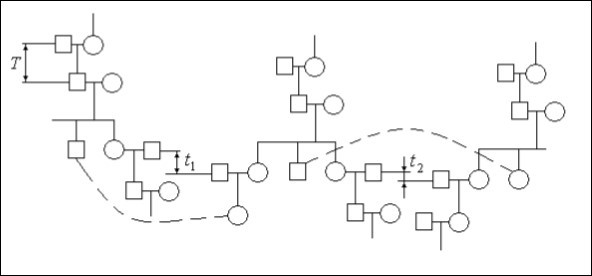For an example three family trees are shown. The dotted line shows possible ways of crossing of male and female individuals in family trees. If for a family tree it is possible to consider the time of one alternation generation approximately T≈25 - 30 years for a population this time can be any t1, t2, etc. For a population continuous alternation of generations is characteristic. Therefore a family tree and a population from the point of view of mathematical genetics laws should be analyzed differently.

The purpose of given article is attempt of mathematical modeling of mutational processes evolution in a population.

## Populational Dynamics of Genome at Discrete Alternation of Generations

Before to proceed to studying influence of the mutational factor to a population we shall consider some mathematical questions of genetic inheritance in case of this factor absence influence.

For a family tree the analysis we shall carry out on the basis of Hardy – Weinberg law 3. This law in the elementary kind of two alleles of a gene establishes that relative frequencies of genotypes in generations of a family tree correspond to members of binomial expansion (p + q)2 so p + q = 1 where p and q frequencies of a dominant A and recessive a alleles in a family tree. It is supposed that a modeling family tree large enough so it is possible inbreeding to neglect. Besides there exists panmixia, is no selection, mutations, migrations of individuals, etc. 1.

According to this law genotypesАА,Аа, andаа at autosomal inheritance have the following frequency ratio:

(AA)p2: (Aa)2pq: (aa)q2 …...(1)

The Hardy - Weinberg equilibrium state is indifferent. On the autosomal inheritance it is obvious. Really, using distribution of genotypes (1) it is possible to receive, for example, the frequency of a recessive allele a in the following (n + 1) generation. For this purpose it is necessary for the previous generation n to do summation of the half of heterozygotes frequencyАа, and the homozygotes frequencyаа: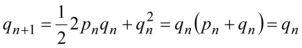……...(2)

In the following (n + 1) generation there is same frequency of a recessive allele a as in the previous n generation received.

For the linked to a sex genome the analysis is necessary more complex.

Let's assume that alleles A and a are linked to the Х-chromosome. The frequency of a dominant allele A in the men is pm and in the women pf . For recessive allele a it is accordingly qm and qf.

At crossing in the first generation there is a ratio of genotypes in the women according to product (pf + qf) (pm +qm):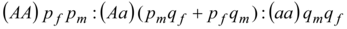……(3)

Using the distribution of genotypes (3) it is possible to find a frequency of allele a in the women for the following (n + 1) generation:…..(4)

In the deduction (4) the following obvious ratios pmn = 1-qmn, and pfn = 1-qfn are used.

Thus, the frequency of allele a in the women for the following generation is equal to a half-sum of the allele frequencies a in women and man of the previous generation between whom there was a reproductive contact. The formula (4) can be copied in the following kind: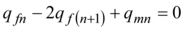........(5)

For convenience of the formula (5) further analysis we shall write down it with displacement on one generation back: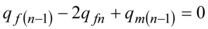.........(6)

At absence of mutagen effect the frequency of allele a at men is equal to frequency of this allele at women of the previous generation qm(n-1) = qf(n-2). Using the given condition, from (6) we shall find: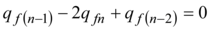.........(7)

The solution of the finite-differential equation (7) we search as qfn = bn where in this caseb is constant. Substituting this solution in the formula (7) we have: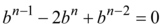……...(8)

Let's divide the equation (8) on b n-2:

b - 2b2 + 1= 0 ……..(9)

We find two roots of the characteristic quadratic (9):

b1=1, and b2=1/2, …..(10)

Hence the general solution of the finite-differential equation (7) looks like:

qfn= C1 + C2(-1/2)n …….(11)

The formula (11) has been found by a different way in 4.

Constants of integration c1 also c2 we shall find on the basis of the initial conditions: at n=0 , q fn=q f0, and at n = 1 according to (4) qfn = qf1 = qmo + qf0 / 2. Thus:

C1 = 2qf0 + qm0 /3, and C2 = qf0 + qm0 / 3. ….(12)

Therefore the solution (11) finally looks like: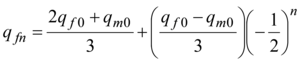.......(13)

On figure 2 the dependence of a recessive allele a frequency linked with the Х-chromosome in a family tree at the women from the number of generation n plotted under the formula (13) is shown.

The initial conditions for calculation are accepted qf0 = 0.6, and qm0 = 0.2. The figure 2 shows how a recessive allele a frequency at women during crossings comes nearer to the equilibrium frequency qfn = 2q f0 + q m0 / 3 = 0.467.

## Populational Dynamics of Genome at Continuous Alternation of Generations

Hardy - Weinberg law in the form before considered concerns to a separate family tree. Implicitly this law includes time because alternation of generations occurs through certain time. Thus Hardy - Weinberg law has the discrete character on time. The population will consist of family trees crossed among themselves and lives in continuous time. Alternation of generations of the family trees set results to generations are replaced actually according to a continuous time scale.

In it the basic distinction of the Hardy - Weinberg law for a population and a family tree. In earlier used form (1) Hardy - Weinberg law is written down for a family tree.

Let's pass to a continuous scale of the generations n. Under size n in this case we mean time t lives of the population normalized on average on a population time of a one generation life, i.e. actually continuous dimensionless time n = t/T.

Let's find out, whether there is the differential equation having the characteristic equation similar (8).

For this purpose we shall consider the differential equation:

…….(14)

where Ƞ is constant. In this formula qf(n-1) there is recessive allele frequency at the woman in n - 1 generation. Differentiation in (14) goes on dimensionless time n.

Let's transform the equation (14) to finite-differential form: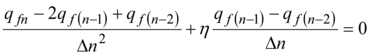…….(15)

where size Δn there is the time step of finite-differential representation of a derivative.

Uniting similar members and multiplying the equation (15) on - 2 we shall find: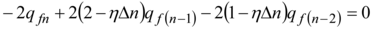......(16)

Let's try to identify the equations (16) and (7) for alternation of the recessive allele frequencies in generations of a family tree. For this purpose it is necessary to accept:

2(2 - ȠΔn) = 1, and -2(1 - ȠΔn) = 1 …….(17)

Wonderful feature of the algebraic equations (17) is that they have the identical solution:

ȠΔn = 3/2 …...(18)

It’s means that the finite-differential equation (7) and the differential equation (14) can have the same characteristic equation. Taking into account (18) the equation (14) can be copied as: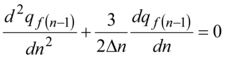……..(19)

The equation (19) can be integrated once: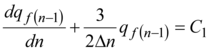……..(20)

where C1 is constant of integration.

Further integrating the equation (20) by method of a separation of variables:

…….(21)

Where q fo there is initial frequency of examined recessive allele at the woman we shall find qfn= qf(n) - the time dependence of it allele on frequency: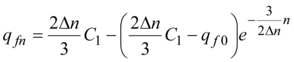……..(22)

Identifying the solution (22) with the solution (13) we shall find:

and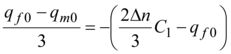……..(23)

Where qm0 there is initial frequency of examined recessive allele at the man.

As it was expected the formulas (23) do not contradict each other. Hence, the solution (22) can be written down as:.........(24)

The formula (24) is correct for frequency of female allele a only in even generations. This is consequence of transition to the continuous scale of the time n.

Comparing (13) and (24) for even generations we have 2-n=e-3/2Δn nor Δn = 3/2ln 2 . Hence (24) it will be transformed to the kind:

…...(25)

that is identical to the formula (13) for even generations n. But in (25) as against (13) the size n is a dimensionless time. Numbers of generation in (25) are not present.

Taking into account (18), and Δn = 3/2ln 2 we shall find Ƞ=ln 2. Thus the differential equation (19) will be written down as:

........(26)

As generations in a population are replaced continuously in the formula (26) there is no necessity to specify number of generation. The formula (26) represents Hardy - Weinberg law for a population. We shall note that Hardy - Weinberg law in form (26) as well as (1) reflects indifferent balance of a genome since to it satisfies the solution qf = const.

On figure 3 dependence plotted under the formula (25) of allele a frequency linked withthe Х-chromosome in a population at women from dimensionless time n is shown at the same initial conditions as dependence on figure 2. Equilibrium frequency of allele there is same as well as in case of discrete alternation of generations in a family tree.

Figure 2. Dependence of a recessive allele a frequency in a family tree at women from the number of generation n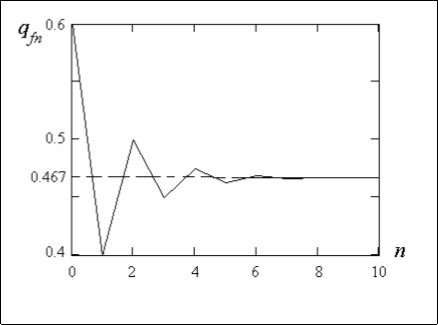Figure 3. Dependence of a recessive allele a frequency in a population at women on dimensionless time n=t/T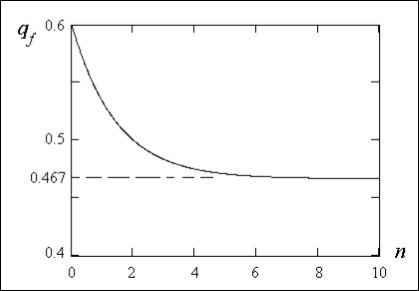## Mutational Influence on a Population

Let's consider influence of some mutational factor on a population. It can be abrupt change of the environment characteristics: increase of ultra-violet or radioactive radiation, emission of harmful products of a natural (volcanic) or artificial origin, distribution of pesticides, etc. All these factors change of the alleles frequency in a population, i.e. cause genic modification.

It is possible to result as an example decrease in frequency of the recessive alleles of the genes determining the concentration of a catalase in blood in 15.5 times approximately for 2 generations for people living in Hiroshima and Nagasaki in comparison with other people of Japan. Herewith the frequency of the dominant alleles practically has not changed 5.

The characteristic of a system response on some influence generally is Green's function of this system 6. Therefore first of all we shall find Green's function for a population.

Let's consider the differential equation (26) reflecting the Hardy – Weinberg law for female allele linked with a sex for a population on which some mutational factor operates influencing only on a recessive allele a:

……..(27)

Where D (n) there is function of time describing influence of the mutational factor on a population.

Let's search the solution of the equation (27) as: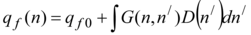…….(28)

where q f0 there is initial (before the beginning of influence on a population) frequency of recessive allele in the woman, G(n, n| ) - Green's function for a population, n| - the parameter of integration – time (moment) of mutational influence the response on which is investigated in time (moment) n, so n > n/ .

Let's substitute the formula (28) in the equation (27):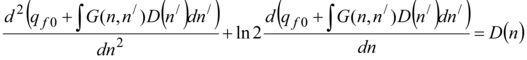...........(29)

Let's accept the following initial conditions reflecting that initial frequency of a recessive allele does not depend on time .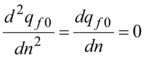In this case the equation (29) will be transformed to a kind: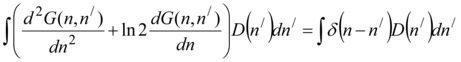………...(30)

Where ẟ (n - n/) there is Dirac’s function.

In (30) we used known property of Dirac’s ẟ - function 7: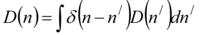……….(31)

where Dirac’s ẟ - function has the following determination ẟ (n - n/) = 0 at n ≠ n|, and ẟ (n - n|) = ∞ at n = n/.

Thus from the equation (30) we find the equation for Green's function of a population:

.........(32)

The linear differential equation of the second order (32) easily is solved by introduction of a new variable.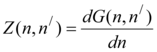Let's note that Green's function of a population does not depend on a kind of influence. Green's function of a population is a characteristic of a population but of not the influence kind on it. It shows how the population reacts to conditional instant mutational influence as ẟ -function in the initial moment of time n - n| .

Further assuming Z=uv we find the function v=e-n ln 2. For function u the differential equation looks like:

......(33)

which solution is: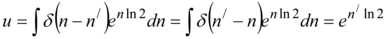......(34)

In a finding (34) we are used the evenness of the ẟ-function and property (31). Hence there is. Z=uv=e-(n-n| )ln2. Green's function of a population find by a condition: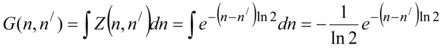.....(35)

Formula (35) can be presented as: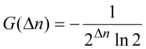…….(36)

where Δn = n-n| there is time interval from the moment of influence n| .

The sign a minus specifies that the population always resists to the external influence.

On figure 4 the change of Green’s function of a population in case of the elementary two-allele system linked to a sex is shown. The minimal value of the Green’s function at Δn = 0 it is equal G(Δn) =-1.443. On Δn→ ∞ the Green's function is to tend to zero. Thus the response of a population to mutational influence it is gradual disappearance of an influence result. Actually, already in 4 - 5 generation a genome of a population comes back to an initial level which existed before the influence. Due to crossing the mutations as are soluble in a population. This fact is well-known to selectors. The cultural plants are given themselves quickly become natural.

Figure 4. Green’s function for the two-allele genomic system linked to a sex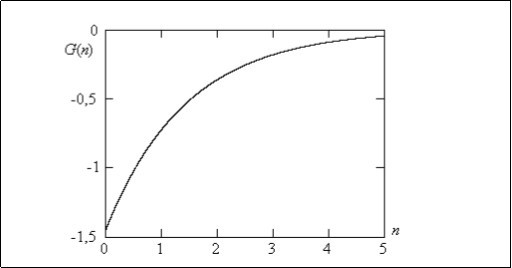Let's substitute Green's function (36) in the formula (28):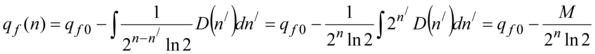………..(37)

wherethere is parameter of a mutation, and integration is carried out on all dimensionless time of the mutational factor influence from 0 up to n|max. We shall notice that as against the equation for Green's function (32) in the formula (37) time of influence not instant.

Thus the law (37) of a recessive allele frequency change at women in a population in response to mutational influence carries universal on time n character. Depending on a kind of mutational process changes only the parameter of a mutation M dependent also from duration of mutational process n|max.

For example if mutational influence is carried out with the help of gradually radioactive element decay, D(n|)=D(0)2-αn|8, where α=T/T1/2 , and T1/2 there is half-life of a radioactive element. Hence the parameter of a mutation is equal:….(38)

The elementary formula for parameter of a mutation occurred if the half-life of a radioactive element is equal to average time of generation alternation, i.e. α=1. In this case the parameter of a mutation linearly depends on time of mutational influence M=D(0)n|max. For example to this condition for the person satisfy half-life of such widespread radioactive elements, as caesium Cs13755 - 30.2 years, strontium Sr9038 - 28.7 years 9 though it is usual half-life of radioactive elements much less.

If the radioactive doze is proportional to time of influence the linear dependence of parameter of a mutation on time of influence well correlates with the experimental linear curve “doze – effect” for Drosophila melanogaster3. Biophysical mechanisms of mutations at a radioactive irradiation are considered in 10.

## Conclusion

The populations consist of family trees set which individuals are crossed among themselves. At mathematical research of the family trees genotypes distribution it is necessary to use Hardy - Weinberg law in the discrete form, and at research of a population in the form of the differential equation of the second order.

The characteristic of a population reaction on mutational influence is Green's function. It shows how the population reacts to instant mutational influence as Dirac’s ẟ-function.

The response of the population genic system on mutational influence is levelling of this influence due to crossing individuals. The population comes back to an initial level for 4-5 generations.

The law of a recessive allele frequency change in a population in reply to mutational influence has universal character on time.